# Count-min sketch in Java with Examples

• Last Updated : 27 Jun, 2021

The Count-min sketch is a probabilistic data structure. The Count-Min sketch is a simple technique to summarize large amounts of frequency data. Count-min sketch algorithm talks about keeping track of the count of things. i.e, How many times an element is present in the set. Finding the count of an item could be easily achieved in Java using HashTable or Map
Trying MultiSet as an alternative to Count-min sketch
Let’s try to implement this data structure using MultiSet with the below source code and try to find out issues with this approach.

Attention reader! Don’t stop learning now. Get hold of all the important DSA concepts with the DSA Self Paced Course at a student-friendly price and become industry ready.  To complete your preparation from learning a language to DS Algo and many more,  please refer Complete Interview Preparation Course.

In case you wish to attend live classes with experts, please refer DSA Live Classes for Working Professionals and Competitive Programming Live for Students.

## java

 `// Java program to try MultiSet as an``// alternative to Count-min sketch` `import` `com.google.common.collect.HashMultiset;``import` `com.google.common.collect.Multiset;` `public` `class` `MultiSetDemo {``    ``public` `static` `void` `main(String[] args)``    ``{``        ``Multiset blackListedIPs``            ``= HashMultiset.create();``        ``blackListedIPs.add(``"192.170.0.1"``);``        ``blackListedIPs.add(``"75.245.10.1"``);``        ``blackListedIPs.add(``"10.125.22.20"``);``        ``blackListedIPs.add(``"192.170.0.1"``);` `        ``System.out.println(blackListedIPs``                               ``.count(``"192.170.0.1"``));``        ``System.out.println(blackListedIPs``                               ``.count(``"10.125.22.20"``));``    ``}``}`

Output: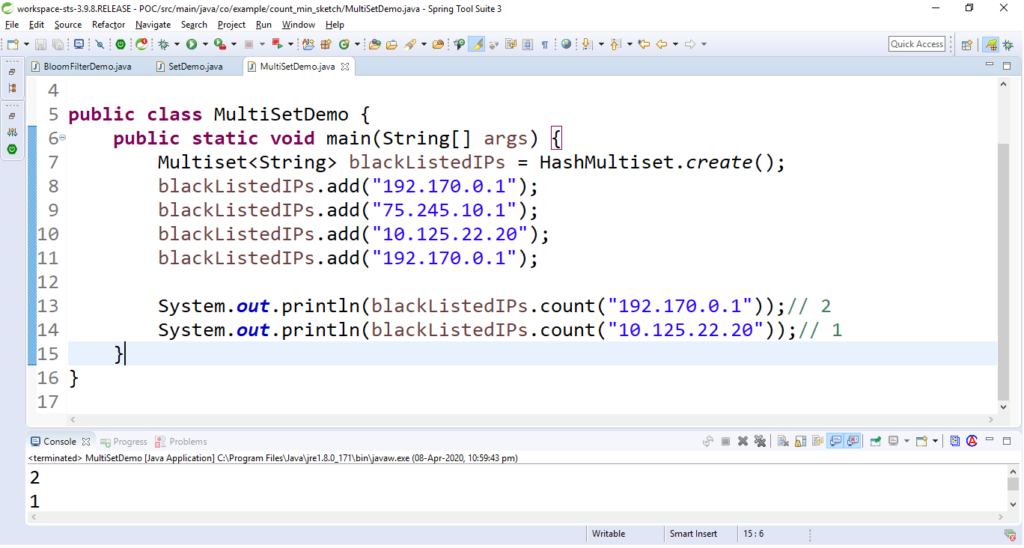Understanding the problem of using MultiSet
Now let’s look at the time and space consumed with this type of approach.

```----------------------------------------------
|Number of UUIDs          Insertion Time(ms)  |
----------------------------------------------
|10                         <25               |
|100                        <25               |
|1, 000                      30               |
|10, 000                     257              |
|100, 000                    1200             |
|1, 000, 000                 4244             |
----------------------------------------------```

Let’s have a look at the memory (space) consumed:

```----------------------------------------------
|Number of UUIDs            JVM heap used(MB) |
----------------------------------------------
|10                         <2                |
|100                        <2                |
|1, 000                      3                |
|10, 000                     9                |
|100, 000                    39               |
|1, 000, 000                 234              |
-----------------------------------------------```

We can easily understand that as data grows, the above approach is consuming a lot of memory and time to process the data. This can be optimized if we use count-min sketch algorithm.
What is Count-min sketch and how does it work?
Count-min sketch approach was proposed by Graham Cormode and S. Muthukrishnan. in the paper approximating data with the count-min sketch published in 2011/12. Count-min sketch is used to count the frequency of the events on the streaming data. Like Bloom filter, Count-min sketch algorithm also works with hash codes. It uses multiple hash functions to map these frequencies on to the matrix (Consider sketch here a two dimensional array or matrix).
Let’s look at the below example step by step.

1. Consider below 2D array with 4 rows and 16 columns, also the number of rows is equal to the number of hash functions. That means we are taking four hash functions for our example. Initialize/mark each cell in the matrix with zero.
Note: The more accurate result you want, the more number of hash function to be used.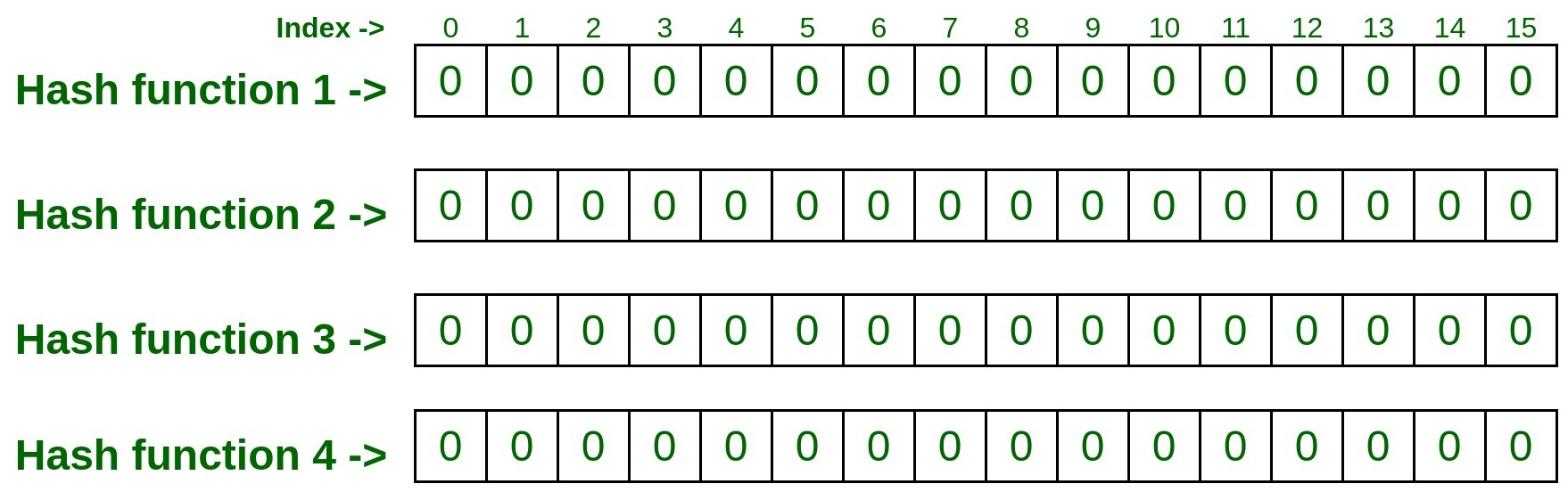1.
2. Now let’s add some element to it. To do so we have to pass that element with all four hash functions which will may result as follows.

`Input : 192.170.0.1 `
1.
```hashFunction1(192.170.0.1): 1
hashFunction2(192.170.0.1): 6
hashFunction3(192.170.0.1): 3
hashFunction4(192.170.0.1): 1```
1. Now visit to the indexes retrieved above by all four hash function and mark them as 1.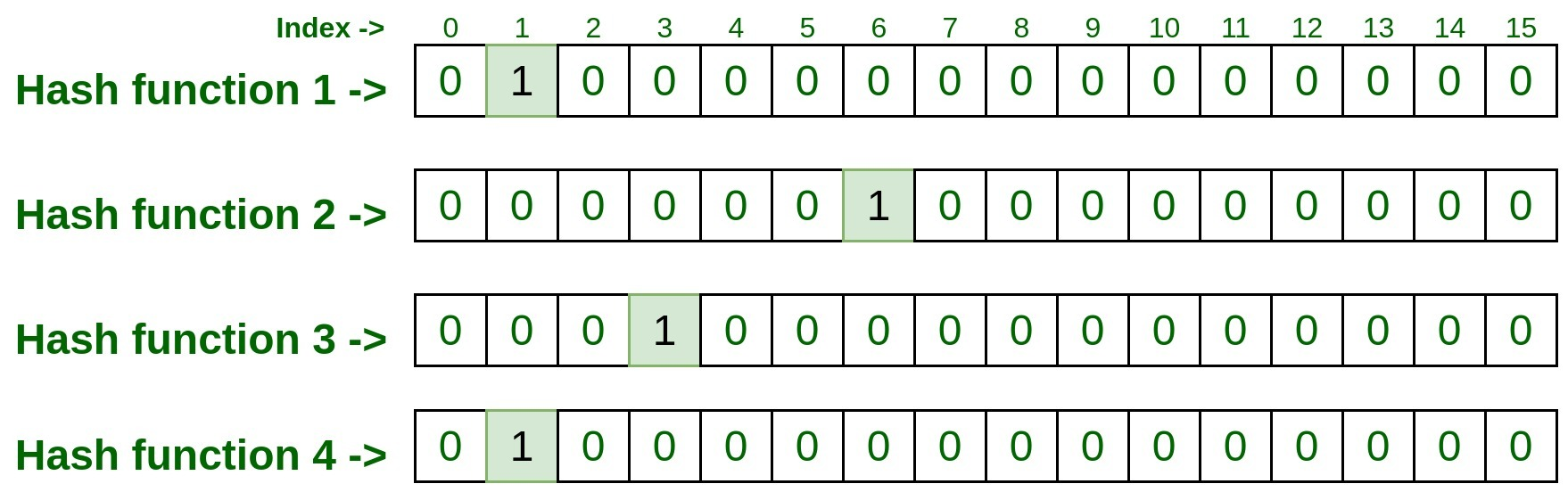1.
2. Similarly process second input by passing it to all four hash functions.

`Input : 75.245.10.1 `
1.
```hashFunction1(75.245.10.1): 1
hashFunction2(75.245.10.1): 2
hashFunction3(75.245.10.1): 4
hashFunction4(75.245.10.1): 6```
1. Now, take these indexes and visit the matrix, if the given index has already been marked as 1. This is called collision which means that, the index of that row was already marked by some previous inputs and in this case just increment the index value by 1. In our case, since we have already marked index 1 of row 1 i.e., hashFunction1() as 1 by previous input, so this time it will be incremented by 1 and now this cell entry will be 2, but for rest of the index of rest rows it will be 0, since there was no collision.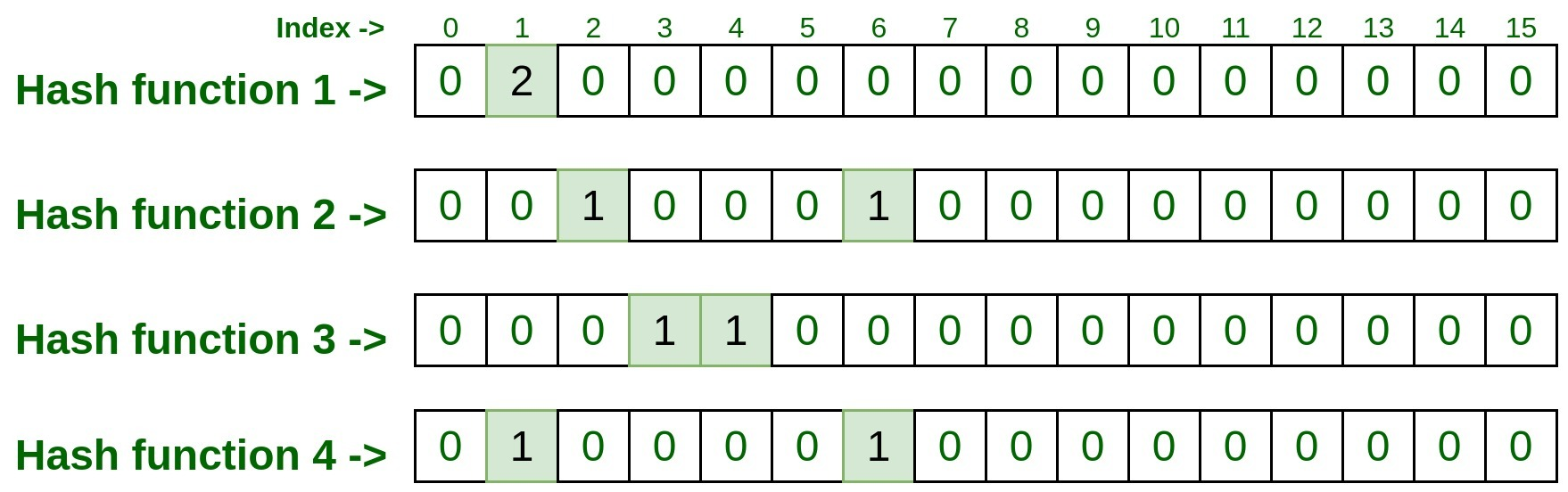1.
2. Let’s process next input

`Input : 10.125.22.20 `
1.
```hashFunction1(10.125.22.20): 3
hashFunction2(10.125.22.20): 4
hashFunction3(10.125.22.20): 1
hashFunction4(10.125.22.20): 6```
1. Lets, represent it on matrix, do remember to increment the count by 1 if already some entry exist.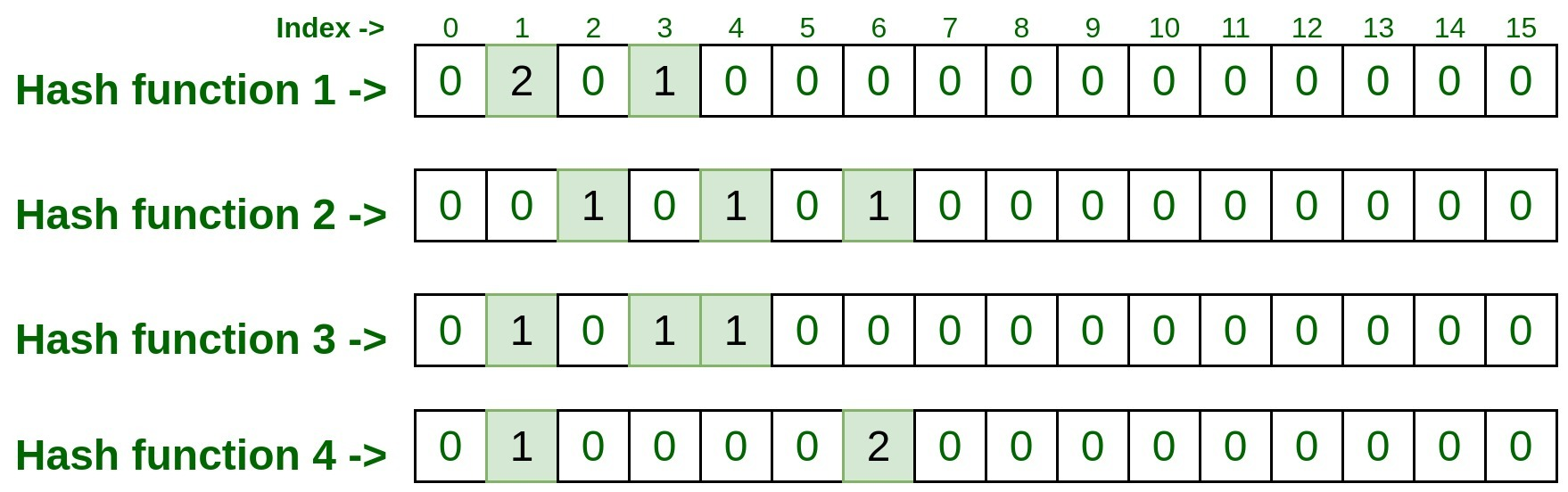1.
2. Similarly process next input.

`Input : 192.170.0.1 `
1.
```hashFunction1(192.170.0.1): 1
hashFunction2(192.170.0.1): 6
hashFunction3(192.170.0.1): 3
hashFunction4(192.170.0.1): 1```
1. Let’s have a look on matrix representation.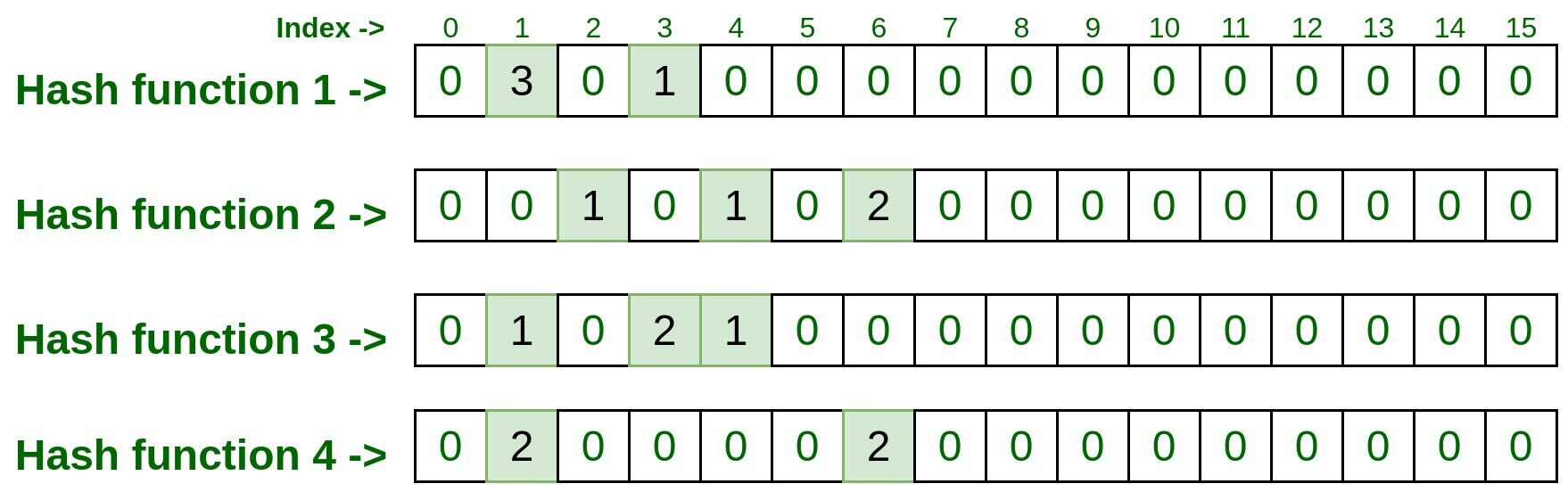1.

Now let’s test some element and check how many time are they present.

`Test Input #1: 192.170.0.1 `
1. Pass above input to all four hash functions, and take the index numbers generated by hash functions.

```hashFunction1(192.170.0.1): 1
hashFunction2(192.170.0.1): 6
hashFunction3(192.170.0.1): 3
hashFunction4(192.170.0.1): 1```
1. Now visit to each index and take note down the entry present on that index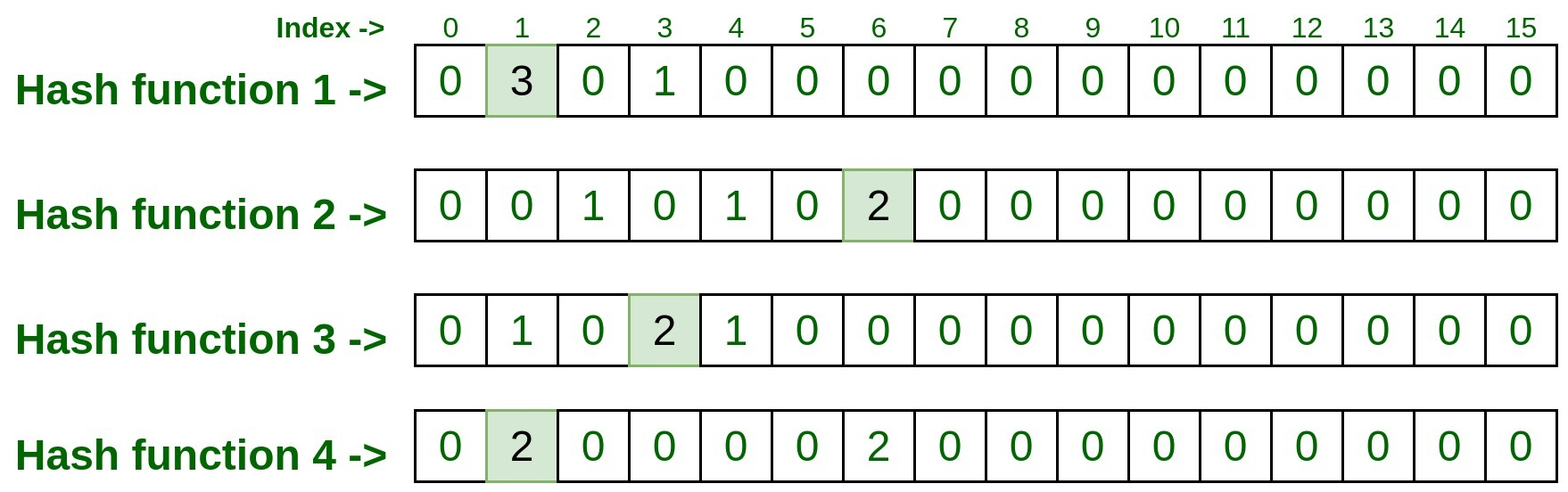1. So the final entry on each index was 3, 2, 2, 2. Now take the minimum count among these entries and that is the result. So min(3, 2, 2, 2) is 2, that means the above test input is processed two times in the above list.

`Test Input #1: 10.125.22.20 `
1. Pass above input to all four hash functions, and take the index numbers generated by hash functions.

```hashFunction1(10.125.22.20): 3
hashFunction2(10.125.22.20): 4
hashFunction3(10.125.22.20): 1
hashFunction4(10.125.22.20): 6```
1. Now visit to each index and take note down the entry present on that index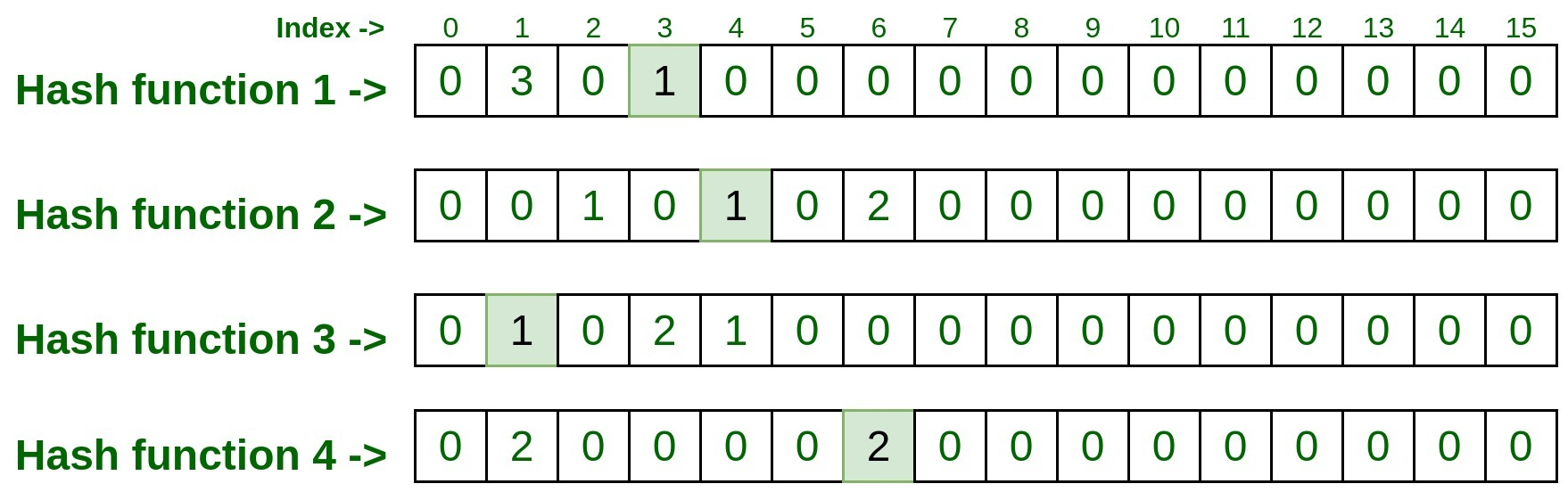1. So the final entry on each index was 1, 1, 1, 2. Now take the minimum count among these entries and that is the result. So min(1, 1, 1, 2) is 1, that means the above test input is processed only once in the above list.

Issue with Count-min sketch and its solution:
What if one or more elements got the same hash values and then they all incremented. So, in that case, the value would have been increased because of the hash collision. Thus sometimes (in very rare cases) Count-min sketch overcounts the frequencies because of the hash functions. So the more hash function we take there will be less collision. The fewer hash functions we take there will be a high probability of collision. Hence it always recommended taking more number of hash functions.
Applications of Count-min sketch:

• Compressed Sensing
• Networking
• NLP
• Stream Processing
• Frequency tracking
• Extension: Heavy-hitters
• Extension: Range-query

Implementation of Count-min sketch using Guava library in Java:
We can implement the Count-min sketch using Java library provided by Guava. Below is the step by step implementation:

1. Use below maven dependency.

## XML

 `<``dependency``>``    ``<``groupId``>com.clearspring.analytics``    ``<``artifactId``>stream``    ``<``version``>2.9.5```
1.
2. The detailed Java code is as follows:

## Java

 `import` `com.clearspring.analytics``    ``.stream.frequency.CountMinSketch;` `public` `class` `CountMinSketchDemo {``    ``public` `static` `void` `main(String[] args)``    ``{``        ``CountMinSketch countMinSketch``            ``= ``new` `CountMinSketch(``                ``// epsilon``                ``0.001``,``                ``// delta``                ``0.99``,``                ``// seed``                ``1``);` `        ``countMinSketch.add(``"75.245.10.1"``, ``1``);``        ``countMinSketch.add(``"10.125.22.20"``, ``1``);``        ``countMinSketch.add(``"192.170.0.1"``, ``2``);` `        ``System.out.println(``            ``countMinSketch``                ``.estimateCount(``                    ``"192.170.0.1"``));``        ``System.out.println(``            ``countMinSketch``                ``.estimateCount(``                    ``"999.999.99.99"``));``    ``}``}`
1.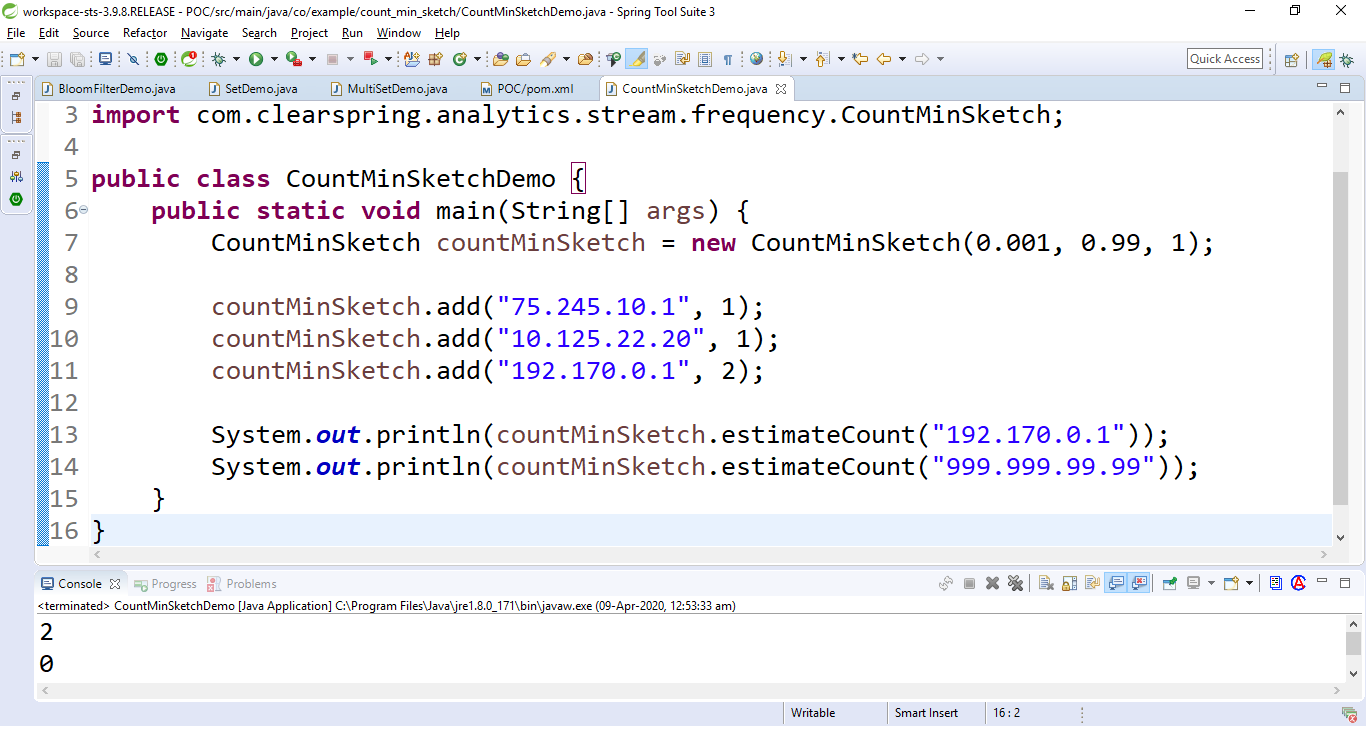1.
2. Above example takes three arguments in the constructor which are

```- 0.001 = the epsilon i.e., error rate
- 0.99 = the delta i.e., confidence or accuracy rate
- 1 = the seed```
1.
2. Now lets have a look at the time and space consumed with this approach.

```-----------------------------------------------------------------------------
|Number of UUIDs | Multiset Insertion Time(ms)  | CMS Insertion Time(ms)    |
-----------------------------------------------------------------------------
|10                         <25                   35                        |
|100                        <25                   30                        |
|1, 000                      30                   69                        |
|10, 000                     257                  246                       |
|100, 000                    1200                 970                       |
|1, 000, 000                 4244                 4419                      |
-----------------------------------------------------------------------------```
1.
2. Now, Let’s have a look of the memory consumed:

```--------------------------------------------------------------------------
|Number of UUIDs | Multiset JVM heap used(MB) | CMS JVM heap used(MB)    |
--------------------------------------------------------------------------
|10                         <2                   N/A                     |
|100                        <2                   N/A                     |
|1, 000                      3                   N/A                     |
|10, 000                     9                   N/A                     |
|100, 000                    39                  N/A                     |
|1, 000, 000                 234                 N/A                     |
---------------------------------------------------------------------------```
1.
2. Suggestions:

```-------------------------------------------------------------------------------------
|Epsilon  | Delta  | width/Row (hash functions)| Depth/column| CMS JVM heap used(MB) |
-------------------------------------------------------------------------------------
|0.1      |0.99    |         7                 | 20          |   0.009               |
|0.01     |0.999   |         10                | 100         |   0.02                |
|0.001    |0.9999  |         14                | 2000        |   0.2                 |
|0.0001   |0.99999 |         17                | 20000       |   2                   |
--------------------------------------------------------------------------------------```

Conclusion:
We have observed that the Count-min sketch is a good choice in a situation where we have to process a large data set with low memory consumption. We also saw that the more accurate result we want the number of hash functions(rows/width) has to be increased.

My Personal Notes arrow_drop_up